﻿ C : Insert a new node at the middle of the Linked List

# C Exercises: Insert a new node at the middle of the Linked List

## C Singly Linked List : Exercise-6 with Solution

Write a program in C to insert a node in the middle of a Singly Linked List.

Pictorial Presentation: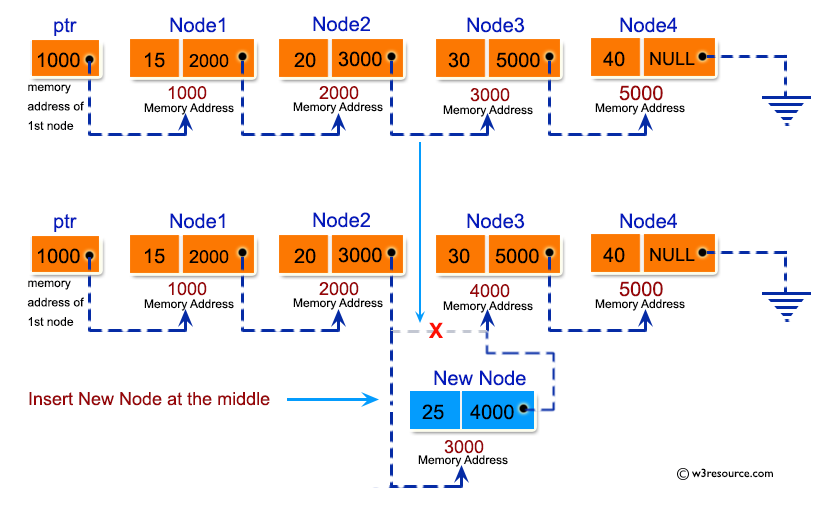Sample Solution:

C Code:

``````#include <stdio.h>
#include <stdlib.h>

struct node
{
int num;                    //Data of the node
struct node *nextptr;       //Address of the node
}*stnode;

void createNodeList(int n);     				//function to create the list
void insertNodeAtMiddle(int num, int pos);	    //function to insert node at the middle
void displayList();             				//function to display the list

int main()
{
int n,num,pos;
printf("\n\n Linked List : Insert a new node at the middle of the Linked List :\n");
printf("-----------------------------------------------------------------------\n");

printf(" Input the number of nodes (3 or more) : ");
scanf("%d", &n);
createNodeList(n);
printf("\n Data entered in the list are : \n");
displayList();
printf("\n Input data to insert in the middle of the list : ");
scanf("%d", &num);
printf(" Input the position to insert new node : " );
scanf("%d", &pos);
if(pos<=1 || pos>=n)
{
printf("\n Insertion can not be possible in that position.\n ");
}
if(pos>1 && pos<n)
{
insertNodeAtMiddle(num, pos);
printf("\n Insertion completed successfully.\n ");
}
printf("\n The new list are : \n");
displayList();
return 0;
}
void createNodeList(int n)
{
struct node *fnNode, *tmp;
int num, i;
stnode = (struct node *)malloc(sizeof(struct node));
if(stnode == NULL) //check whether the stnode is NULL and if so no memory allocation
{
printf(" Memory can not be allocated.");
}
else
{
// reads data for the node through keyboard
printf(" Input data for node 1 : ");
scanf("%d", &num);
stnode-> num = num;
tmp = stnode;
for(i=2; i<=n; i++)
{
fnNode = (struct node *)malloc(sizeof(struct node));
if(fnNode == NULL) //check whether the fnnode is NULL and if so no memory allocation
{
printf(" Memory can not be allocated.");
break;
}
else
{
printf(" Input data for node %d : ", i);
scanf(" %d", &num);

fnNode->num = num;      // links the num field of fnNode with num
fnNode->nextptr = NULL; // links the address field of fnNode with NULL

tmp->nextptr = fnNode; // links previous node i.e. tmp to the fnNode
tmp = tmp->nextptr;
}
}
}
}

void insertNodeAtMiddle(int num, int pos)
{
int i;
struct node *fnNode, *tmp;
fnNode = (struct node*)malloc(sizeof(struct node));
if(fnNode == NULL)
{
printf(" Memory can not be allocated.");
}
else
{
fnNode->num = num; //Links the data part
fnNode->nextptr = NULL;
tmp = stnode;
for(i=2; i<=pos-1; i++)
{
tmp = tmp->nextptr;

if(tmp == NULL)
break;
}
if(tmp != NULL)
{
tmp->nextptr = fnNode;
}
else
{
printf(" Insert is not possible to the given position.\n");
}
}
}

void displayList()
{
struct node *tmp;
if(stnode == NULL)
{
printf(" No data found in the empty list.");
}
else
{
tmp = stnode;
while(tmp != NULL)
{
printf(" Data = %d\n", tmp->num);   // prints the data of current node
tmp = tmp->nextptr;                 // advances the position of current node
}
}
}
```
```

Sample Output:

```Linked List : Insert a new node at the middle of the Linked List :
-----------------------------------------------------------------------
Input the number of nodes (3 or more) : 4
Input data for node 1 : 1
Input data for node 2 : 2
Input data for node 3 : 3
Input data for node 4 : 4

Data entered in the list are :
Data = 1
Data = 2
Data = 3
Data = 4

Input data to insert in the middle of the list : 5
Input the position to insert new node : 3

Insertion completed successfully.
The new list are :
Data = 1
Data = 2
Data = 5
Data = 3
Data = 4
```

Flowchart: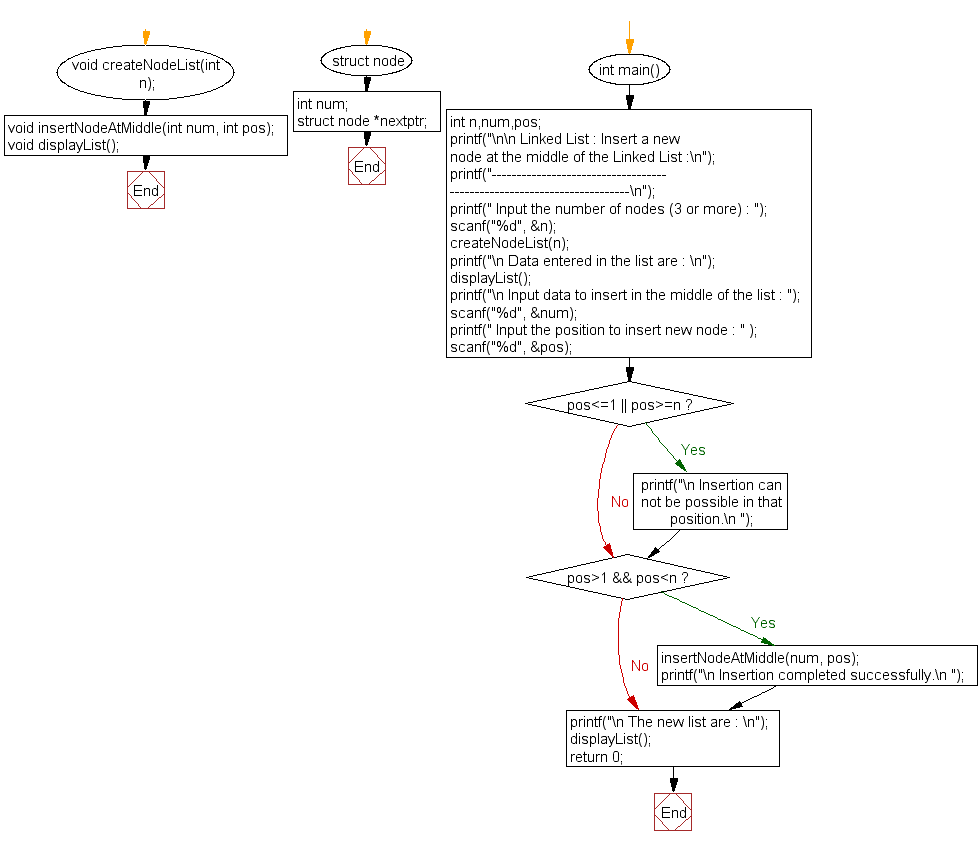createNodeList():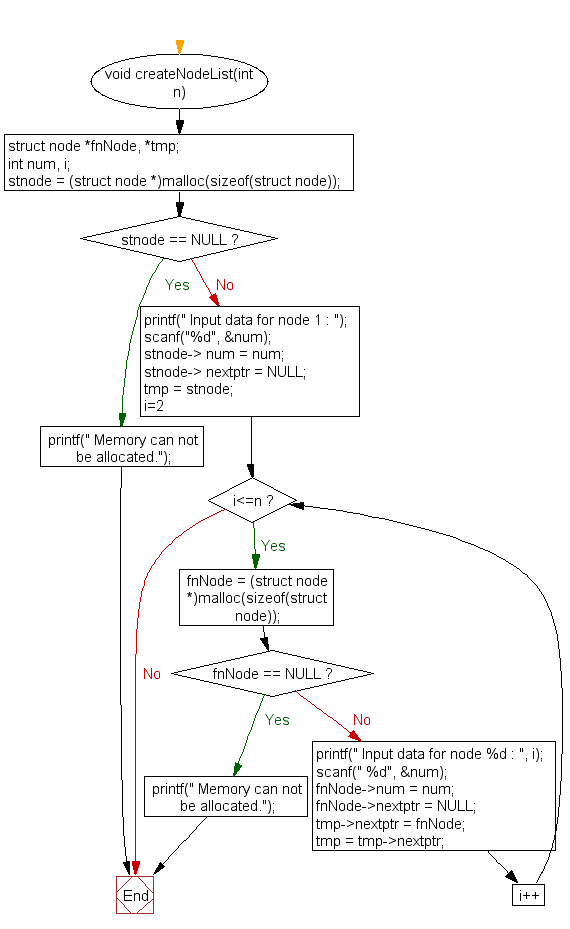insertNodeAtMiddle() :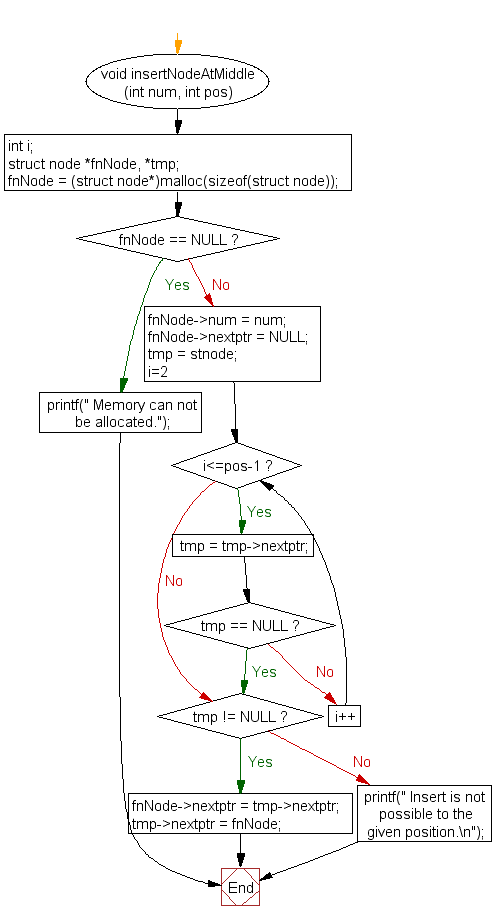displayList() :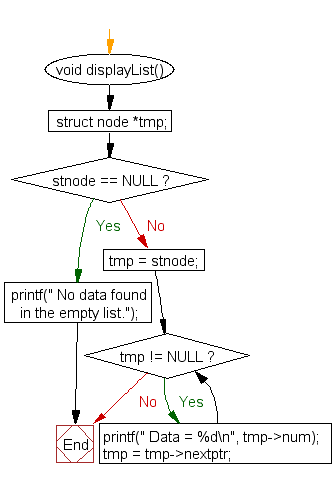C Programming Code Editor:

What is the difficulty level of this exercise?

Test your Programming skills with w3resource's quiz.

﻿